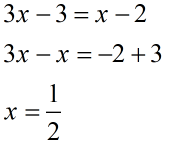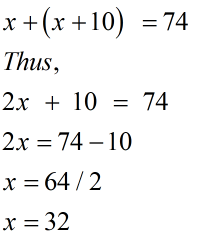• Call Now

1800-102-2727•

# NCERT Solutions for Class 8 Maths Chapter 2- Linear Equations in One Variable: Download Free PDFClass 8 Maths Chapter 2: The NCERT chapter manages the equations with linear expressions in a single variable, known as Linear Equations in One Variable. This exercise has 65 questions incorporating various concepts of the solution, a portion of its applications, decreasing equations to a less complex structure and word problems identified with Linear Equations in One Variable.

## Class 8 Maths Chapter 2- Linear Equations in One Variable- Important Topics

Solving Linear Equations
Performing Mathematical Operations on Equations: While we perform mathematical operations on a linear condition, we ought to do it on the two sides of the equality that won't remain constant.

Solving Equations with variables on both sides
The equation 3x - 3 = x – 2, has variables on both of its sides. Step 1: Transpose all the terms with a variable from the right-hand side to the left-hand side of the mentioned equation and all the constants from the left-hand side to the right-hand side of the equation. 3x – x = -2+3 Step 2: Divide the two sides of the equation by the coefficient of the Variable. ⇒ x = 1/2Applications (Word Problems)
Sum of two numbers is 74. If a number exceeds the other by 10, then what are the numbers? Let the numbers be x and x+10 Given that the sum of the two is 74, Therefore, x+(x+10) =74 Thus, 2x + 10 = 74 ⇒2x =74−10 ⇒x = 64/2 ⇒x = 32 The numbers are 32 and 42, respectively.Variables and Constants
A constant is a certain value or number that never shows signs of change in an expression, and it's constantly the same. A variable is a letter representing some obscure value.

Algebraic Equation
The assertion of equality of two algebraic articulations is an algebraic equation, such as P=Q, where P and Q are algebraic articulations. 6x + 5 and 5x + 3 are algebraic articulations by equating which, we get an algebraic equation. 6x + 5 = 5x + 3 is an algebraic equation.

Linear Equations in One Variable
A linear equation is a certain algebraic equation where each term is either a constant or its product and a single variable with 1 as its highest power. A linear equation with a single variable is called a Linear Equation in One Variable.

## FAQs for NCERT Solutions For Class 8 Maths

QWhy are NCERT solutions for Class 8th essential?

A. NCERT solutions provide topic-wise solutions of the chapters, which helps the student to prepare for their exams and understand each topic nicely. The basic concepts are cleared by providing step by step methods. Mathematics of class 8 is fundamental and challenging, so it is essential to have a solution handy. Aakash even provides some new methods and techniques to solve the problems which makes the studies easier. There are a lot of Question papers, teaching materials, and online books written by Aakash’s various teachers across the country. The whole purpose of teaching is to prepare the students for their exams as well as for their future.

QHow do I find the Aakash NCERT solutions?

A. You can find NCERT Solutions from the Aakash website. The solutions are arranged in chapter-wise and topic-wise order. They can be found in a pdf format.

QHow to score good marks in the internal assessments of Mathematics in the 8th grade?

A. The best way to score marks in Mathematics would be to practise. Just going through the solutions for the NCERT PDF provided by Aakash won’t help you to the same level. However, if you practise the questions using the Aakash NCERT solutions, then it will help you drastically to score excellent marks in the internal assessments for class 8th Mathematics.

Q.Where and how can a student download free PDF latest NCERT solutions for class 8 for all subjects?

A. For the ease of students, we at Aakash have provided a PDF file for NCERT Solutions of all subjects, all topics for all classes. The detailed solutions are prepared by our senior and experienced faculty which give students an in-depth understanding of the concepts rather than the particular question.

Q. Why Choose Aakash for Class 8 NCERT Solutions?

A. The solutions provided by us are prepared by IITians and well-trained faculty members. Thus, they are reliable, precise and give the best techniques to solve any problem. We also include some tips to enable students to solve problems with speed and accuracy.

## NCERT Class 8 Maths Solutions - Chapterwise Free PDFs for Download### NCERT Solutions For Other Class

Talk to our expert

By submitting up, I agree to receive all the Whatsapp communication on my registered number and Aakash terms and conditions and privacy policy